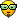Expansion of the C-Gear Table/creating formulas

Forum for collaboration projects in Algodoo Phun Edition.

Expansion of the C-Gear Table/creating formulas

#Teeth|#Circles|Ratio|Number|Rotation(degrees)
6 | 12 | 2/9 | 4.5 | 30 |
12 | 24 | 1/9 | 9 | 15 |mother ratio (ratio I used to make this table)
24 | 48 | 1/18| 18 | 7.5
36 | 72 | 1/27| 27 | 5
48 | 96 | 1/36| 36 | 3.75
60 | 120 | 1/48| 48 | 3.5

Use the save "How to make better gears"

Here is as far as I got (in the middle of class)
explanation
In the construction of a c gear
you usually put circles ALL around and then delete every other circle in the end...
you can also use every other circle in some sort of double gear with collision layers
if you just wanted every other circle DOUBLE THE ANGLE

Angle: the rotation you use before putting on each circle
Teeth: the amount of circles you have after you delete every other circle
Circles: full saturated amount on BIG circle
Number: multiplicationally(personally I beleive thats a real word) ratio between BIG circle and tooth(or circle,small ones)
Ratio: fractional ratio between tooth and BIG circle

I beleive in 7th grade they teach you a process of creating fractional equivalents...
math lesson for ALL ages(like baby genius/child progidies XD)
2/4 --> ?/2
1 2 3 4

2/4 --> x4/2
1 2 3 4
cross multiply
numbers
1 and 4
2*2=4
divide
product with number 2
4/4=1
2/4 == 1/2

Story:I was trying to create my own C-gear, and made a 12-tooth c-gear with a 0.81 BIG circle 0.09 tooth circle
rotating 15 degrees and deleting every other circle.
I though the secret with a 12-tooth was with exponents VIA-squaring 0.09squared is 0.81
then I tried cubing ans stuff
After some mumbo jumbo I though of using ratios. and BUDAM!
And that, Charlie Brown, is how the C-Gear Table was born
ITS NOT EXPONENTS ITS RATIOS!!!!!!

current formula base I made
9(number),12(tooth),24(circles) 18 (number)
-- ---- --- ---
15(angle) 15 15 30 (thirty times nine divided by fifteen) (do the same to the other values, use the same denominator

just fractional equivolate
if you want to specify a value, just put it below
like if you wanted a 28 tooth, put the angle on the top
and tooth below, mass fractional-equivolate the numbers
just use one specification.
to make a fractional ratio with the number jsut put a one and dash on top of it
1
--
9
Project: Develope a bigger table and formulae(instead of justt fractional equivolations) to be used in the expansion of the table and creation of C-Gears
For ALL of Algodonia(aka Algodoo)-may the geek be with you
GMtester

Posts: 4
Joined: Sun May 24, 2015 8:12 pm